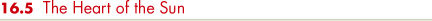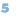Compared with familiar terrestrial events—storms, tidal waves, tornadoes, even violent volcanic eruptions and earthquakes—the spots and flares of the active Sun are enormously energetic. The much greater steady emission from the inactive quiet Sun simply staggers the imagination. The Sun is somehow able to produce huge amounts of energy, and according to Earth's fossil record, it has been doing so for the last several billion years. What powers the Sun? What forces are at work in the Sun's core to produce such energy? By what process does the Sun shine, day after day, year after year, eon after eon? Answers to these questions are central to all astronomy. Without them, we can understand neither the physical existence of stars and galaxies in the universe nor the biological existence of life on Earth.

SOLAR ENERGY PRODUCTION

In round numbers, the Sun's luminosity is 4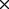1026 W and its mass is 21030 kg. We can quantify how efficiently the Sun generates energy by dividing the solar luminosity by the solar mass: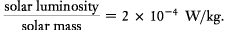This simply means that, on average, every kilogram of solar material yields about 0.2 milliwatts of energy—0.0002 joules (J) of energy every second. This is not much energy—a piece of burning wood generates about a million times more energy per unit mass per unit time than does our Sun. But there is an important difference: the wood will not burn for billions of years.

To appreciate the magnitude of the energy generated by our Sun, we must consider not the ratio of the solar luminosity to the solar mass but instead the total amount of energy generated by each gram of solar matter over the entire lifetime of the Sun as a star. This is easy to do. We simply multiply the rate at which the Sun generates energy by the age of the Sun, about 5 billion years. We obtain a value of 31013 J/kg. This is the average amount of energy radiated by every gram of solar material since the Sun formed. It represents a minimum value for the total energy radiated by the Sun, for more energy will be needed for every additional day the Sun shines. Should the Sun endure for another 5 billion years (as is predicted by theory), we would have to double this value.

This energy-to-mass ratio value is very large. At least 60 trillion joules (on average) of energy must arise from every kilogram of solar matter to power the Sun throughout its lifetime. But the generation of energy is not explosive, releasing large amounts of energy in a short period of time. Instead, it is slow and steady, providing a uniform and long-lived rate of energy production. Only one known energy-generation mechanism can conceivably power the Sun in this way. That process is nuclear fusion—the combining of light nuclei into heavier ones.

NUCLEAR FUSION

We can represent a typical fusion reaction symbolically as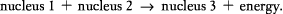For powering the Sun, the most important piece of this equation is the energy produced. Where does it come from?

The key point is that during a fusion reaction, the total mass decreases—the mass of nucleus 3 is less than the combined masses of nuclei 1 and 2. To understand the consequences of this, we use a very important law of modern physics—the law of conservation of mass and energy. Albert Einstein showed at the beginning of the twentieth century that matter and energy are interchangeable. One can be converted into the other, in accordance with Einstein's famous equation, E = mc2. To determine the amount of energy corresponding to a given mass, simply multiply it by the square of the speed of light (c in the equation). For example, the energy equivalent of 1 kg of matter is 1(3108)2, or 91016 J. The speed of light is so large that even small amounts of mass translate into enormous amounts of energy.

The law of conservation of mass and energy states that the sum of mass and energy (properly converted to the same units) must always remain constant in any physical process. There are no known exceptions. According to this law, an object can literally disappear, provided that some energy appears in its place. If magicians really made rabbits disappear, the result would be a flash of energy equaling the product of the rabbit's mass and the square of the speed of light—enough to destroy the magician, everyone in the audience, and probably all the surrounding state as well! In the case of fusion reactions in the solar core, the energy is produced primarily in the form of electromagnetic radiation. The light we see coming from the Sun means that the Sun's mass must be slowly but steadily decreasing with time.

THE PROTON—PROTON CHAIN

All atomic nuclei are positively charged, so they repel one another. Furthermore, by the inverse-square law, the closer two nuclei come to one another, the greater is the repulsive force between them.(Sec. 3.2) How then do nuclei—two protons, say—ever manage to fuse into anything heavier? The answer is that if they collide at high enough speeds, one proton can momentarily plow deep into the other, eventually coming within the exceedingly short range of the strong nuclear force, which binds nuclei together (see More Precisely 16-1). At distances of less than about 10-15 m, the attraction of the nuclear force overwhelms the electromagnetic repulsion, and fusion occurs. Speeds in excess of a few hundred kilometers per second, corresponding to a gas temperature of 107 K or more, are needed to slam protons together fast enough to initiate fusion. Such conditions are found in the core of the Sun and at the centers of all stars.

The fusion of two protons is illustrated schematically in Figure 16.25. In effect, one of the protons turns into a neutron, creating new particles in the process, and combines with the other proton to form a deuteron, the nucleus of a special form of hydrogen called deuterium. Deuterium (also referred to as "heavy hydrogen") differs from ordinary hydrogen by virtue of an extra neutron in its nucleus. We can represent this reaction as follows: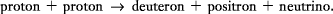The positron particle in this reaction is a positively charged electron. Its properties are identical to those of a normal negatively charged electron, except for its positive charge. Scientists call the electron and the positron a "matter—antimatter pair"—the positron is said to be the antiparticle of the electron. These newly created positrons find themselves in the midst of a sea of electrons, with which they interact immediately and violently. The particles and antiparticles annihilate one another, producing pure energy in the form of gamma-ray photons.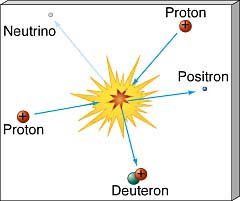Figure 16.25 Two protons collide violently, initiating the chain of nuclear fusion that powers the Sun.

The final product of the reaction is a particle known as a neutrino, a word derived from the Italian for "little neutral one." Neutrinos carry no electrical charge and are of very low mass—at most 1/100,000 the mass of an electron, which itself has only 1/2000 the mass of a proton. (The exact mass of the neutrino is uncertain, although recent evidence strongly suggests that it is not zero.) They move at (or nearly at) the speed of light and interact with hardly anything. They can penetrate, without stopping, several light years of lead. Their interactions with matter are governed by the weak nuclear force, also described in More Precisely 16-1. Despite their elusiveness, however, neutrinos can be detected with carefully constructed instruments (Section 16.6).

Nuclei such as normal hydrogen and deuterium, containing the same number of protons but different numbers of neutrons, represent different forms of the same element—they are known as isotopes of that element. Usually, there are about as many neutrons in a nucleus as protons, but the exact number of neutrons can vary, and most elements can exist in a number of isotopic forms. To avoid confusion when talking about isotopes of the same element, nuclear physicists attach a number to the symbol representing the element. This number indicates the total number of particles (protons plus neutrons) in its nucleus. Ordinary hydrogen is denoted by 1H, deuterium by 2H. Normal helium (2 protons plus 2 neutrons) is 4He (also referred to as helium-4), and so on. We will adopt this convention for the rest of this book. We can now rewrite the preceding proton—proton reaction asThis equation is labeled (I) because the production of a deuteron by the fusion of two protons is the first step in the fusion process powering most stars. It is the start of the proton-proton chain. Gargantuan quantities of protons are fused in this way within the core of the Sun each second. The positrons produced by this reaction quickly encounter electrons and are annihilated, releasing energy in the form of gamma rays.

The next step in solar fusion is the formation of an isotope of helium. A proton interacts with the deuteron particle produced in step (I):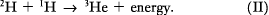This step begins as soon as deuterons appear. The reaction product is an isotope of helium—helium-3 (3He)—lacking one of the neutrons contained in the normal helium-4 nucleus. Energy is also emitted, again in the form of gamma-ray photons.

The third and final step in the proton—proton chain involves the production of a nucleus of helium-4. Helium-4 (4He) comes about most often through the fusion of two of the helium-3 nuclei created in step (II):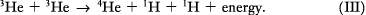The result is a helium-4 nucleus plus two more protons.

The net effect of steps (I) through (III) is this: four hydrogen nuclei (protons; six consumed, two returned) combine to create one helium-4 nucleus, plus some gamma-ray radiation and two neutrinos. The whole process is illustrated in Figure 16.26. Symbolically, we have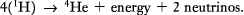The gamma rays are slowly degraded in energy as they pass through the solar interior. Photons are repeatedly absorbed by electrons and ions, then reemitted at a wavelength that reflects the temperature of the surrounding gas, in accordance with Wien's law.(Sec. 3.4) Thus, as the radiation makes its way to the surface through ever-cooler layers, its wavelength decreases. Eventually, it leaves the surface in the form of visible light. The neutrinos escape unhindered into space. The helium stays put in the core.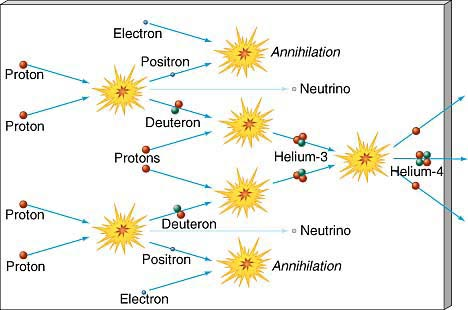Figure 16.26 Diagram of the entire proton—proton chain. A total of six protons (and two electrons) are converted into two protons, one helium-4 nucleus, and two neutrinos. The two leftover protons are available as fuel for new proton—proton reactions, so the net effect is that four protons are fused to form one helium-4 nucleus. Energy, in the form of gamma rays, is produced in each reaction.

This basic process is responsible for the light emitted by almost all the stars we see. Alternative reactions leading to the same final result exist (see More Precisely 20-1), but they are relatively rare in stars like the Sun.

ENERGY GENERATED BY THE PROTON—PROTON CHAIN

Now let's calculate the energy produced in the fusion process and compare it with the 61013 J/kg needed to account for the Sun's luminosity. Careful laboratory experiments have determined the masses of all the particles involved in the conversion of four protons into a helium-4 nucleus: the total mass of the protons is 6.694310-27 kg, the mass of the helium-4 nucleus is 6.646610-27 kg, and the neutrinos are virtually massless. The difference between the total mass of the protons and the helium nucleus is 0.04810-27 kg—not much mass, but easily measurable. Multiplying the vanished mass by the square of the speed of light yields 4.310-12 J—this is the energy produced by the fusion of 6.710-27 kg (the rounded-off mass of the four protons) of hydrogen into helium. It follows that fusion of 1 kg of hydrogen would generate 6.41014 J—more than enough to power the Sun, even for another 5 billion years. To fuel the Sun's present energy output, hydrogen must be fused into helium in the core at a rate of 600 million tons per second.